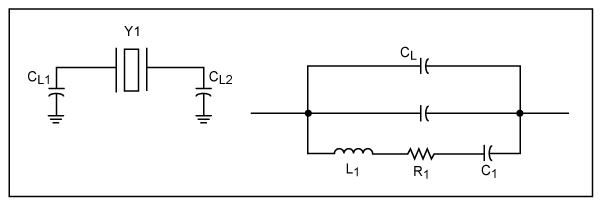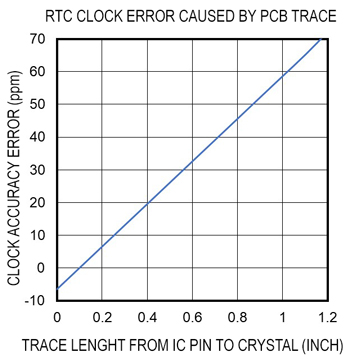# Guidelines on the Effects of Parasitic Capacitance on Clock Accuracy Due to Board Layout

### Abstract

Crystal oscillator is widely used in RTC applications. Clock accuracy, one of the key parameters in real-time clocks, partly depends on the parasitic capacitance of the PCB being employed. Optimizing PCB layout can achieve the desired clock accuracy.

### Introduction

In a crystal-based real-time clock (RTC) system, the mismatch between the actual and specified capacitance load of the crystal can create frequency errors, resulting in degradation of clock accuracy. While crystals and RTC ICs have been well defined and designed, the uncertainty is in the printed circuit board (PCB) design. Users often have a dilemma on how to implement the PCB design to obtain the specified clock accuracy. The rule of thumb is to follow the PCB design of its evaluation kit (EV kit) as closely as possible since the RTC IC has been trimmed and characterized based on its EV kit. However, in real system design, it is not practicable to copy the EV kit PCB exactly. Therefore, a trade-off needs be considered between clock accuracy, PCB stackup and PCB layout.

### Crystal Oscillator Basics

Using a crystal oscillator to provide a fundamental frequency, 32.768kHz, is a popular approach in RTC IC designs. The basic block diagram of a crystal oscillator is shown in Figure 1, where crystal capacitance loads CL1 and CL2 are usually implemented inside the RTC ICs with an external crystal.

The crystal load capacitance looking from two terminals is:

CL = (CL1 × CL2)/(CL1 + CL2) + CSTRAY

where CSTRAY is the additional capacitance from the PCB trace and the mounting pads of crystal and RTC IC.

Usually a crystal is specified at a certain capacitance load that it expects to see, such as 6.0pF or 12.5pF, so that it will operate at the specified frequency. If the load differs from the specification, a frequency error occurs and causes a clock accuracy error.Figure 2 presents the equivalent circuit of a crystal and the load it expects to see.With an external load capacitance (CL), the crystal oscillator oscillates at the frequency based on the equation below.Where,

FL = Oscillation frequency with capacitance CL
CT = Overall effective capacitance looking from two crystal terminals with CL
CT = C1 × (CL + C0)/(CL + C0 + C1)
FS = Series resonate frequency of the crystalThe above equations define the relationship between oscillating frequency and capacitance load. Further derivation with approximation can obtain the frequency variation with capacitance load CL in ppm/pF.Equation 1. Crystal oscillator frequency shift with load capacitance change ppm per pF.

The formula in Equation 1 is only valid near the specified CL region. When CL gets bigger and bigger, the impact to clock frequency is less and less, but it is an appropriate estimation in this discussion.

### Clock Error with PCB Layout

Variation in oscillation frequency of the cystal oscillator is desirable in some applications, such as VCOs. However, in RTC systems, this causes clock inaccuracy. Maxim Integrated RTC products typically have load capacitors CL1 and CL2 on-chip in RTC IC production, these two load capacitors are trimmed to yield the optimal clock accuracy based on EV kit PCB layout. In other words, the stray capacitance in the EV kit is included as part of CL1 and CL2. If the PCB layout is offset from the EV kit, the RTC cannot achieve the specified clock accuracy. We can simulate and calculate clock errors, in regard to CL change, with trace length and PCB dielectric thickness between crystal pads and ground.

Typical crystal parameters used in RTCs are as follows:

C0 = 1.3pF
C1 = 0.007pF
CL = 6.0pF

Placing the above crystal parameters into Equation1, the clock error is roughly equal to 65ppm per pF change in CL.

In Maxim Integrated RTC EV kits, typically the PCB stackup is 0.062 inches per two-layer PCB with FR4 dielectric material. The trace between IC pins and crystal is about 0.1 inches, with a crystal mounting pad of about 0.025 x 0.045 inches.

Simulation shows the stray capacitance of the trace is about 1.04 per inch.

The simulated stray capacitance adding to CL in the EV kit is 0.2pF. This capacitance is already included in the IC production trim for CL1 and CL2 to yield the specified clock accuracy.In real applications, it is not always feasible to use a two-layer PCB due to increased system complexity. Sometimes the top layer, which accommodates the RTC IC and crystal, only has 4ml separation from the second ground layer. In this case, the stray capacitance changes and causes a discrepancy in clock accuracy.

The stray capacitance with ground layer height shows in Table 1 and Figure 3 below.

 Height from Crystal to Ground (ml) Simulated Stray Capacitance Change (pF) Clock Error(ppm) 60 0 0 30 0.06 3.9 10 0.2 13 5 0.45 29.25 4 0.62 40.3### Summary

RTC clock accuracy is significantly affected by PCB trace and board stackup. Users should pay close attention to the PCB design in applications where high clock accuracy is required. When the PCB stackup is of multi-layer type, it is recommended that the thickness of the dielectric substrate between the layer with crystal and ground plane is as thick as possible in consideration of clock accuracy. If the PCB stackup must utilize a thin dielectric layer such as 4 or 5mls, due to line impedance or a total board thickness limitation, the ground beneath the crystal pads and traces should be cut out to reduce parasitic capacitance. This may contradict noise reduction concerns, which usually propose placing ground as close to the crystal as possible and not having any signal trace crossover below. The system designers can trade off and balance all of these factors. The clock error curve discussed above provides a basic guideline on clock error vs RTC PCB designs.